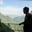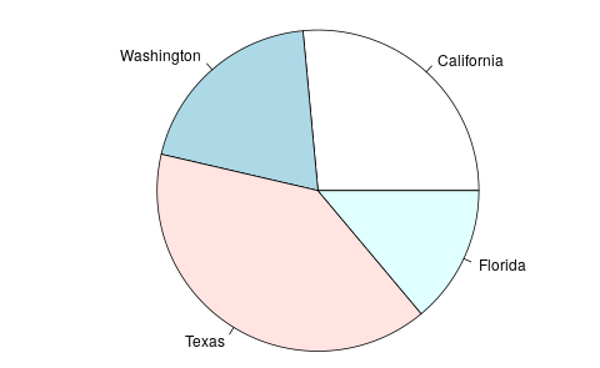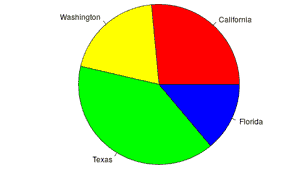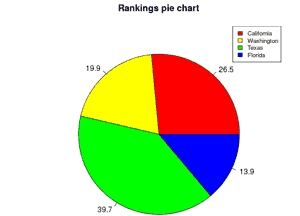Related Tags

r programming
communitycreator
r
pie chart

# How to create pie charts in RAKASH BAJWA

### What are pie charts?

A pie chart is a circular graphical representation that is further subdivided into multiple slices according to the provided numerical values.

### How to create a pie chart in R

There are various libraries available in the R programming language to create different types of graphs. We can make a pie chart in R by using the function pie().

#### pie() function in R

This function accepts positive input values. Various other parameters can be used for this function such as:

• color
• title
• radius
• labels

### Syntax

Let’s discuss the basic syntax of creating a pie chart in R:

Pie(x, labels, radius, main, col, clockwise)


### Parameters

pie() method in R programming accepts the above arguments as above:

• x: shows the positive input values for your chart.

• labels: illustrates the heading or description of each slice of your chart.

• radius: shows the radius of the circle regarding your chart. Its value must be between -1 and +1.

• main: This indicates the title of your graph.

• col: is used for showing the color palette.

• clockwise: we must set a value to draw the pie charts’ slices clockwise or anti-clockwise.

### Code

The image below is the output generated by these lines of code. It takes states ranking x and labels arguments in the pie() method.

# states rankings
x <- c(40, 30, 60, 21)
labels <- c("California", "Washington", "Texas", "Florida")

png(file = "states.png")

# call the function to plot the Pie Chart
pie(x,labels)Simple Pie Chart

### Set colors

To get colors according to each slice, pass the col attribute through the c() method above.

pie(x, labels, col = c("red", "yellow", "green", "blue"))


### Code

This method ranks values, state labels, and multiple colors of slices.

x <- c(40,30,60,21)
labels <- c("California","Washington","Texas","Florida")

# set name for your file
png(file = "states.png")

# call the function to plot the Pie Chart
pie(x,labels,col=c("red","yellow","green","blue"))Pie Chart with Multiple Colors

### Graph legend

The legend illustrates legend keys or different entities on the plotted area of the graph. We can also create a legend for the pie chart by using the following code.

The legend() method in line 9 generates a legend on the pie chart’s top right corner.

# Data for the graph
x <- c(40,30,60,21)

labels <- c("California", "Washington", "Texas", "Florida")
piepercent <- round(100 * x / sum(x), 1)

png(file = "states.png")

# call the function to plot the Pie Chart
pie(x, labels = piepercent,
main = "Rankings pie chart",
col = c("red", "yellow", "green", "blue"))

legend("topright",
c("California", "Washington", "Texas", "Florida"),
cex = 0.8,
fill = c("red", "yellow", "green", "blue"))Pie Cart With Legend (Upper Right Corner)

RELATED TAGS

r programming
communitycreator
r
pie chart

CONTRIBUTORAKASH BAJWA
RELATED COURSES

View all Courses

Keep Exploring

Learn in-demand tech skills in half the time